## Equation Wizard

Sunday 29 April 2007 at 5:43 pm | In Articles | 7 Comments

I have an ambivalent attitude to mathematical software. On the one hand, an enthusiastic user of LaTeX both here and in documents and presentations, but, on the other hand, wary of too much reliance on calculators and computers. OneÂ superb maths teacher I know was criticised for not using a computer in his A level class.Â A Chinese student in the class remarked that they didn’t see much benefit in using one and they had only started to use a calculator when they came to this country to study A levels. They have an important point; I recoil when I ask a simple arithmetic question and the student instinctively reaches for their calculator. Worse still is when they use the calculator on their phone and don’t believe me when I tell them the calculator gets it wrong because it doesn’t obey basic mathematical rules. Similarly, I find graphics calculators are too complicated (computer programs are much easier to use to sketch graphs) and I would prefer to teach students how to sketch curves so that they get a feel for the properties of various functions.

Of course there is a place for calculators and mathematical software. Checking my tax would be a pain without a calculator and graphing software for focusing in at what happens near the origin of the graph of functions likeis fascinating. Similarly, if I am marking student work I use mathematical software to check their matrix operations rather than doing them by hand. Some mathematical software is very powerful and expensive but there are free sites like QuickMath which will solve many problems. However, they should really be used to save time or offer insights after the techniques have been taught and understood – practice, practice and practice is often the best way to learn.

So when I was asked by ElasticLogic to review their Equation WizardÂ Â I made it clear that I would be offering an honest opinion of the program that they sent me.

Equation Wizard is a Windows only program that solves real rational equations and simplifies rational expressions (rational means ratios of polynomials). In fact it claims to solve algebraic equations but that is a misnomer as it doesn’t solve equations involving fractional powers or complex coefficients, though it will give some complexÂ roots (for some reason called imaginaryÂ roots in Help). QuickMath does this for free but Equation Wizard’s strength comes from the fact that it will show the working so the user can understand the method behind the solution.

Entry of polynomials is easy using ^ for powers or using buttons or menus or the Ctrl key and the text is previewed in mathematical form as you type, so x^2 becomesand (x^2-1)/2 becomes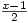; the previewer does its best to interpret ambiguous expressions such as 1/2x. It will add algebraic fractionsÂ showing theÂ working, so if you input 1/(x-1)+1/(x+1) then it is simplified toby adding using a common denominator, multiplying out and collecting the terms in the numerator to get the result, with all steps shown. It uses a similar method to solve the equation 1/(x+1)+1(x+1)=1 finding the answer to 3 decimal places (or up to 9 decimal places if required). There appears to be no limit to the degree of the polynomial equations to be solved – solving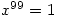was virtually instant.

However, there are limitations. The answers given cannot give exact values so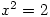gives 1.414 and -1.414 rather than. Rational equations are solved by multiplying by the denominator but the solutions aren’t checked so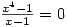gives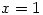as one of four solutions. My Norwegian students were taught to always check their answers, so would know what to do. On the other hand solutions can be missed so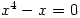gives 0 and 0 as the two solutions, though I expect that’s just a bug. However,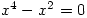gives one correct answer and two wrong ones, which is rather worrying. There are language problems with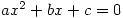being described as a quadric equation. Quadric surfaces are interesting and there are nice pictures here.

You can print out the calculationsÂ or save themÂ in rtf or HTML format (with the equations saved as images) but it would be nice to be able to copy the selected output to the clipboard. Having solved an equation it’s not possible to change it – you have to enter a new one, though you can copy and paste the old one or use the history button, bizarrely labelled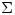.

Your opinions on Equation Wizard are welcome and I hope ElasticLogic will improve this early version as a result of feedback from you. Can you find other equations with missing or incorrect solutions? The software costs \$29 (or Â£15.55 in real money 🙂 ) and you can download a free trial version here.

1. I think once you get to grips with a graphical calculator it’s pretty handy. However it’s a lot of trial and error- in the same way you pick up matlab codes.

I’m quite embarrased that my mental arithmetic is pretty poor due to the fact that in my ‘younger’ years I used to use the calculator all the time. (It’s take me more than a second to work out simple fractions in my head!) 😮

I admit that I instinctively go towards my graphical calculator to draw graphs, however since we’re not allowed using them in the exams I’ve been more controlled.

Oh and I don’t think LaTeX is a maths ‘software’ as such, since it’s a bit like word (don’t shoot me) in the sense that you have to input what you’ve worked out. It doesn’t do it for you.

Sorry for the long comment but colleges nowadays seem to think that it’s important to use technology in class. I mean after we had filled some feedback forms in, my chemistry and further maths teachers started using computers! :/ It was something to do with enhancing learning.

Comment by beans — Sunday 29 April 2007 9:28 pm #

2. On the other hand solutions can be missed so x^4-x=0 gives 0 and 0 as the two solutions, though I expect thatâ€™s just a bug.

The program uses Lobachevsky’s method for finding roots of the equations more than the third degree. It allows to find only the real roots (complex roots are too difficult for programming), with the big lapse. I would be glad, if Steve or blog visitors have advised me a nice method for finding roots (both real and complex) for the equations of the high degrees, which can be easily programmed.

Having solved an equation itâ€™s not possible to change it – you have to enter a new one

For this purpose you can use button “History”.

Comment by ElasticLogic — Sunday 29 April 2007 11:43 pm #

3. I noticed that complex roots aren’t given for higher order polynomials but the problem withis that it misses the real root. Similarly all the roots ofare real but it gives 0, 1.003, 0.997 instead of 0 (twice), -1, 1.

It would be really nice if visitors can help improve the program as requested.

Comment by steve — Monday 30 April 2007 8:54 am #

4. Maybe the program could try finding common factor first…
x^4 – x^2 = 0
x^2(x^2 – 1) = 0

Is that programmable for generic equations? I’m no expert on that.

Comment by Maria — Sunday 6 May 2007 3:15 am #

5. I found there was a steep learning curve. It took a long time to do the problems for the first time. Then after I got the hang of it, it came much quicker.

Ed

Comment by Boulder Math Tutor — Monday 21 May 2007 9:09 pm #

6. If you use the TI-83 or TI-84 calculator, you may find my website useful: TI-83 / TI-84 Programs. Helpful hint: master the technique of your math problem first, then use my programs once that type of math problem becomes tedious and mindless for you.

Comment by Bill Paetzke — Wednesday 30 May 2007 7:27 am #

7. Where are all the good old times when one had to use pencile and paper? I sometimes love too look back – but one also has to go forward. Some of the graphing calculators are amazing. Thin, reliable and intuative that calculator has changed my mind. So I agree with beans that once you have learned how to use them you will never look back.

Comment by Calculator — Saturday 12 September 2009 3:04 pm #

Sorry, the comment form is closed at this time.